# Quotient identities and reciprocal identities

## Quotient and Reciprocal Identities

When it comes to more advanced studies in trigonometry, eventually using just sine, cosine, and tangent on their own won't be enough. That is why it is so important to learn about what we call "quotient" and "reciprocal" identities.

That being said, before we get into using these quotient and reciprocal identities, it is crucial that you have a thorough understanding of how to use sine, cosine, and tangent. Click the links on each of those identities for some quick review on using each of them before you move on in this article. Also, make sure you understand how each of these ratios behaves in a four-quadrant Cartesian Plane by checking out this amazing link here.

## Quotient Identities

In trigonometry, quotient identities refer to trig identities that are divided by each other. There are two quotient identities that are crucial for solving problems dealing with trigs, those being for tangent and cotangent. Cotangent, if you're unfamiliar with it, is the inverse or reciprocal identity of tangent. This identity will be more clear in the next section. Below, this image covers the two fundamental identities you must know when it comes to quotient identities.Quotient Identities

## Reciprocal Identities

Have you ever wondered if there was an easier way of dealing with the trigonometric expressions such as $\sin ^{-1} x$ ? It turns out, there is. In trigonometry, reciprocal identities or inverse identities cover this base. Instead of writing $\sin ^{-1} x$ or $1/ \sin x$ , we can use the reciprocal identity cscx instead. Cosecant (csc), secant (sec), and cotangent (cot) are extremely useful identities, and you will use them extensively as you progress with mathematics into pre-calculus and calculus. Therefore, it is quintessential that you memorize and understand all of these identities. The image below covers what you must know.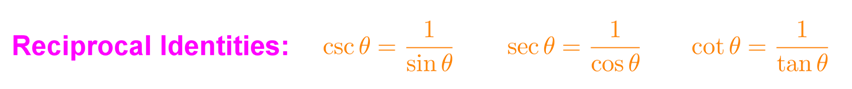Reciprocal Identities

## Using Quotient and Reciprocal Identities

Now that we've covered what quotient and reciprocal identities are, let's get into how to use them. As always, the best way to learn and get comfortable with these identities is to do some practice problems.

Example 1:

Simplify the expression:

$\frac{\cos x \cdot \tan x + \sin x}{2\tan x}$

Step 1: Identify Any Quotient or Reciprocal Identities to Simplify With

In order to simplify this expression, we're definitely going to need to use some trig identities. Notice the presence of tanx on both the numerator and the denominator. Let's replace that with a quotient identity and see if that makes things easier to simplify. The trick with solving these kinds of problems is to try to get everything in the expression in terms of sine and cosine.

$= \frac{\cos x \cdot \frac{\sin x}{\cos x}+ \sin x}{2 \cdot \frac{\sin x}{\cos x}}$

Step 2: Simplify, Simplify, and Simplify Some More

Notice above we can cancel out the cosx's in the numerator. Let's do that and then see if more simplifications can be made.

$=\frac{\sin x + \sin x}{\frac{2 \sin }{\cos x}}$

$= \frac{2 \sin x}{\frac{2 \sin x}{\cos x}}$

$= 2 \sin x \frac{\cos x}{2 \sin x}$

$=\cos x$

And there we have it! Our final answer is cosx. With this example, hopefully you'll begin to realize the power of quotient and reciprocal identities in simplifying trig expressions. This image below gives a visual summary of everything we did to solve this problem: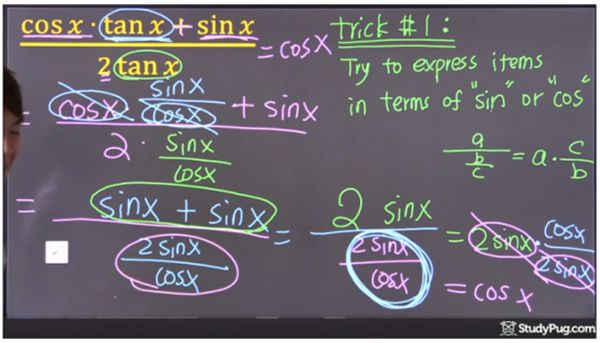Quotient and reciprocal identities example 1 solution

Example 2:

Simplify the expression:

$\frac{\cot x (\sin x + \tan x)}{\csc x + \cot x}$

Step 1: Identify Any Quotient or Reciprocal Identities to Simplify With

In order to simplify this expression, we're definitely going to need to use some trig identities. Notice the presence of several reciprocal and quotient identities we can use on both the numerator and the denominator. Let's replace those and see if that makes things easier to simplify. Again, the trick with solving these kinds of problems is to try to get everything in the expression in terms of sine and cosine.

$= \frac{\frac{\cos x}{\sin x}(\sin x + \frac{\sin x}{\cos x})}{\frac{1}{\sin x}+\frac{\cos x}{\sin x}}$

Step 2: Simplify, Simplify, and Simplify Some More

Now that we've made some substitutions, notice above we can cancel out a lot from the numerator and simplify the fraction in the denominator. Let's do that and then see if more simplifications can be made.

$= \frac{\cos x + 1}{\frac{1+\cos x}{\sin x}}$

$= (\cos x +1) \frac{\sin x}{1+\cos x}$

$= \sin x$

And again, we have successfully simplified this expression! This image below gives a visual summary of everything we did to solve this problem: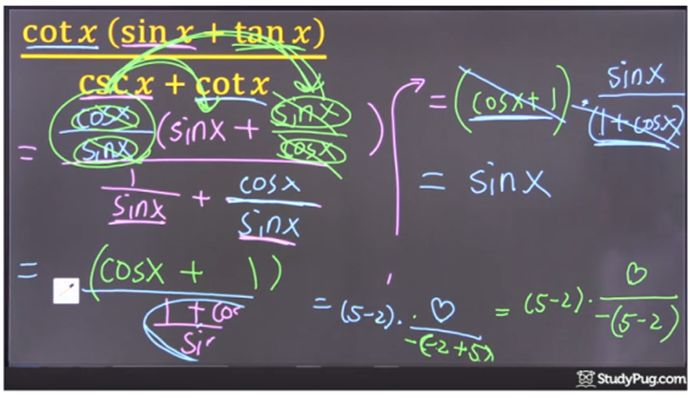Quotient and reciprocal identities example 2 solution

Proving Identities:

The last types of questions you may be asked that deal with quotient and reciprocal identities may be "proof" questions. In these problems, you are commonly asked to "prove" that one side of an equation is equal to the other side of an equation, and you will need to simplify expressions using quotient and reciprocal identities to do so. Let's take a look at an example to see what we mean by "proving identities":

Example:

Prove the following expression is true:

$\frac{\sin A + \tan A}{1 + \cos A} = \frac{1}{1 + \cot A}$

Step 1: Use Quotient and/or Reciprocal Identities to Simplify the Left Hand Side

Just like with what we did in the previous examples, use the power of the quotient and reciprocal identities to simplify the left side as much as you can first.

$\frac{\sin A + \tan A}{1 + \cos A}$

$= \frac{\sin A + \frac{\sin A}{\cos A}}{1+\cos A}$

$= \frac{(\sin A + \frac{\sin A}{\cos A})}{(1 + \cos A)} \cdot \frac{\cos A}{\cos A}$

$= \frac{\sin A \cos A + \sin A}{(1+ \cos A) \cos A}$

$= \frac{\sin A (\cos A +1)}{(1 + \cos A) \cos A}$

$= \frac{\sin A}{\cos A}$

Step 2: Use Identities to Simplify Right Hand Side to Match the Simplified Left Hand Side

Now that we've simplified the left hand side to be a simpler expression, let's try to replicate that expression on the right hand side. If we're successful, we will have successfully solved this problem and made our proof.

$\frac{1}{\cot A}$

$= \frac{1}{(\frac{\cos A}{\sin A})}$

$= 1 \cdot \frac{\sin A}{\cos A}$

$= \frac{\sin A}{\cos A}$

Since the right and left hand sides equal the same expression, we have successfully solved this problem! This image summarizes what we have just accomplished: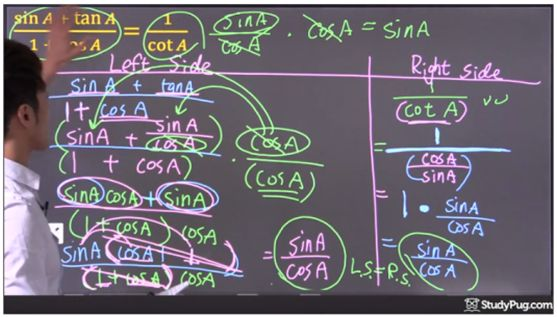Quotient and reciprocal identities example 3 solution

And that's it for quotient and reciprocal identities! It is always handy to have a complete list of trigonometry identities list on hand where you are studying. We have it prepared for you: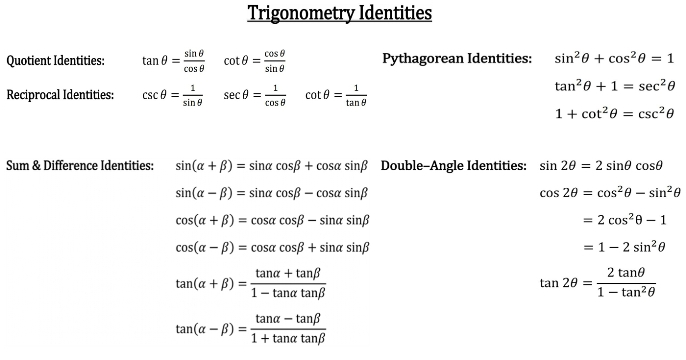Trigonomerty Identities Chart
You can get this excellent trig identities cheat sheet here. In later sections, you are going to learn how to solve trigonometric equations using sum and difference identities. Lastly, if you're looking for how to determine non permissible value for trigs expressions with all of these identities.

### Quotient identities and reciprocal identities

Don't be scared by all the trigonometric identities formulas in this chapter! Dennis will show you how to easily simplify and prove quotient identities and reciprocal identities.

#### Lessons

• 1.
Simplify expressions
a)
$\frac{\cos x \cdot \tan x + \sin x}{2 \tan x }$

b)
$\frac{\cot x (\sin x + \tan x)}{ \csc x + \cot x}$

c)
$\frac{\cos x + \cot x}{1 + \csc x}$

• 2.
Prove identities
a)
$\frac{ \sin A + \tan A}{ 1 + \cos A} = \frac{1}{ \cot A}$

b)
$\frac{\cot x - 1}{1 - \tan x} = \frac{\csc x}{\sec x}$